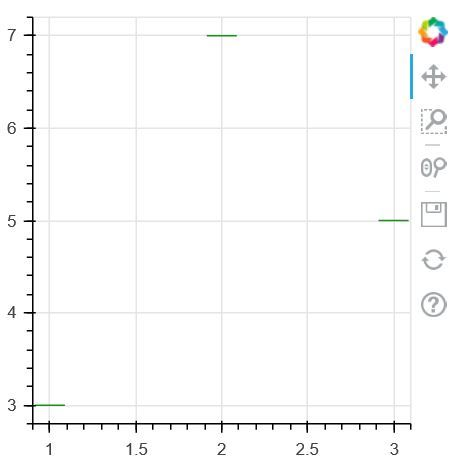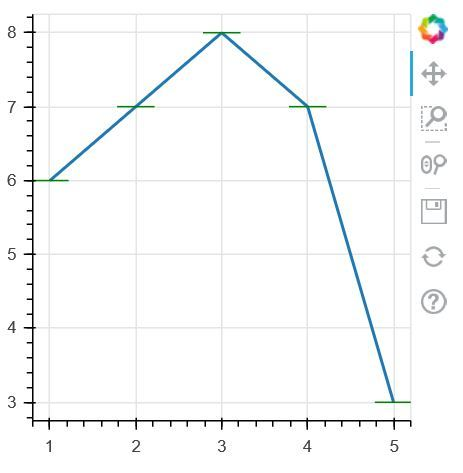Related Articles
bokeh.plotting.figure.dash() function in Python
• Last Updated : 28 Jul, 2020

Bokeh is a data visualization library in Python that provides high-performance interactive charts and plots and the output can be obtained in various mediums like notebook, Html and server. Figure Class create a new Figure for plotting. It is a subclass of Plot that simplifies plot creation with default axes, grids, tools, etc.

## bokeh.plotting.figure.dash() function

The dash() function in the plotting module of bokeh library is used to Configure and add Dash glyphs to this Figure.

Syntax: dash(x, y, size=4, angle=0.0, *, angle_units=’rad’, fill_alpha=1.0, fill_color=’gray’, line_alpha=1.0, line_cap=’butt’, line_color=’black’, line_dash=[], line_dash_offset=0, line_join=’bevel’, line_width=1, name=None, tags=[], **kwargs)
Parameters: This method accept the following parameters that are described below:

• x: This parameter is the x-coordinates for the center of the markers.
• y: This parameter is the y-coordinates for the center of the markers.
• size: This parameter is the size (diameter) values for the markers in screen space units.
• angle: This parameter is the angles to rotate the markers.
• fill_color: This parameter is the fill color values for the markers.
• line_color: This parameter is the line color values for the markers with default value of black.
• line_width: This parameter is the line width values for the markers with default value of 1.
• name: This parameter is the user-supplied name for this model.
• tags: This parameter is the user-supplied values for this model.

Return: This method return the GlyphRenderer value.

Below examples illustrate the bokeh.plotting.figure.dash() function in bokeh.plotting:
Example 1:

## Python3

 `# Implementation of bokeh function  ` `    `  `import` `numpy as np   ` `from` `bokeh.plotting ``import` `figure, output_file, show  ` `    `  `plot ``=` `figure(plot_width ``=` `300``, plot_height ``=` `300``)  ` `plot.dash(x ``=` `[``1``, ``2``, ``3``], y ``=` `[``3``, ``7``, ``5``],   ` `            ``size ``=` `20``, color ``=``"green"``, alpha ``=` `0.9``)  ` `    `  `show(plot)  `

Output:Example 2:

## Python3

 `# Implementation of bokeh function  ` `    `  `import` `numpy as np   ` `from` `bokeh.plotting ``import` `figure, output_file, show  ` `    `  `x ``=` `[``1``, ``2``, ``3``, ``4``, ``5``]  ` `y ``=` `[``6``, ``7``, ``8``, ``7``, ``3``]  ` `   `  `output_file(``"geeksforgeeks.html"``)  ` `   `  `p ``=` `figure(plot_width ``=` `300``, plot_height ``=` `300``)  ` `   `  `# add both a line and circles on the   ` `# same plot  ` `p.line(x, y, line_width ``=` `2``)  ` `p.dash(x, y, fill_color ``=``"red"``,   ` `         ``line_color ``=``"green"``, size ``=` `25``)  ` `   `  `show(p) `

Output:Attention geek! Strengthen your foundations with the Python Programming Foundation Course and learn the basics.

To begin with, your interview preparations Enhance your Data Structures concepts with the Python DS Course.

My Personal Notes arrow_drop_up
Recommended Articles
Page :